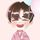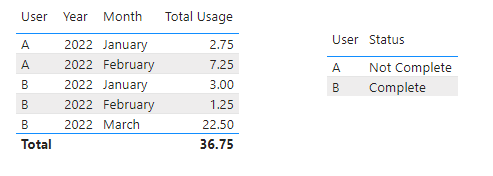cancel
Showing results for
Did you mean:Helper II

## Measure help including circular dependency errors

Hi, Hoping someone can help me create some measures. I'm new to BI and I'm getting circular dependency errors and not sure how to resolve.

I need to show how much time each user is spending in an application per month. A sample of my data source looks like this. The Usage time represents hours.

 User Date Usage Time A 1/8/2022 1.25 A 1/12/2022 1.5 A 2/01/2022 2.5 A 2/21/2022 4.75 B 1/19/2022 3 B 2/12/2022 1.25 B 3/12/2022 1 B 3/19/2022 21.5

I tried the following measure and received a circular dependency error:

Monthly Usage = CALCULATE(SUM('table'[Usage Time]),FILTER(ALL(table[Date]),ENDOFMONTH(table[Date])))

My expected outcome is:

 User Date Usage Time A Jan 2.75 A Feb 7.25 B Jan 3 B Feb 1.25 B Mar 22.5

Once I have this figured out, I need to determine how many users meet the annual requirement of completing 20 hours by year end.
I created this measure: 2022 Goal = "20" (no error)

I created a measure for the difference because we want to measure progress too.
2022 Goal Remaining = CALCULATE([2022 Goal (in hours)]-CALCULATE(SUM('table'[Usage Time]),FILTER(ALL(table[Date]),ENDOFMONTH(table[Date]))))

How would I determine the number of users that have completed the goal vs not complete? In my sample data, User B would show Complete while User A would not. I tried
Status = IF('table'[2022 Goal Remaining]="20","Complete","Not Complete") but received the cirular dependency error.

Any help is appreciated. Thanks!
User900

1 ACCEPTED SOLUTIONCommunity Support

Hi @user900 ,

``Total Usage = CALCULATE(SUM('Table'[Usage Time]),ALLEXCEPT('Table','Table'[User],'Table'[Date].[Year],'Table'[Date].[Month]))``
``````Status =
var _2022Goal = 20
return IF(CALCULATE(SUM('Table'[Usage Time]),ALLEXCEPT('Table','Table'[User]))>=_2022Goal,"Complete","Not Complete")``````

Output:Best Regards,
Eyelyn Qin
If this post helps, then please consider Accept it as the solution to help the other members find it more quickly.

3 REPLIES 3Community Support

Hi @user900 ,

``Total Usage = CALCULATE(SUM('Table'[Usage Time]),ALLEXCEPT('Table','Table'[User],'Table'[Date].[Year],'Table'[Date].[Month]))``
``````Status =
var _2022Goal = 20
return IF(CALCULATE(SUM('Table'[Usage Time]),ALLEXCEPT('Table','Table'[User]))>=_2022Goal,"Complete","Not Complete")``````

Output:Best Regards,
Eyelyn Qin
If this post helps, then please consider Accept it as the solution to help the other members find it more quickly.Helper II

This worked perfectly. Thank you.Super User

@user900 , if you need data of last day of month , try like

Monthly Usage = CALCULATE(SUM('table'[Usage Time]),FILTER(ALL(table[Date]),table[Date] = EOmonth(table[Date],0)))

!! Microsoft Fabric !!
Microsoft Power BI Learning Resources, 2023 !!
Learn Power BI - Full Course with Dec-2022, with Window, Index, Offset, 100+ Topics !!
Did I answer your question? Mark my post as a solution! Appreciate your Kudos !! Proud to be a Super User! !!